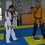# For which $a, b, c$ does the inequality $a^2b+ab^2+b^2c+bc^2+c^2a+ca^2\ge 6abc$ hold?

I know that it holds for non-negatives $a, b, c$ but consider the following:

The inequality $a^3+b^3+c^3\ge 3abc$ holds for non-negatives $a, b, c$ but it holds for real numbers as well iff $(a+b+c)\ge 0$ since: $a^2+b^2+c^2-ab-bc-ca\ge 0 \quad \forall a, b, c \in \mathbb{R} \\ \stackrel{a+b+c\ge 0}{\Longleftrightarrow} (a+b+c)(a^2+b^2+c^2-ac-bc-ca)\ge 0 \\ \stackrel{\text{Euler's Formula}}{\Longleftrightarrow} a^3+b^3+c^3-3abc\ge 0 \\ \Leftrightarrow a^3+b^3+c^3\ge 3abc$ (Generally Note that we can prove the same way that $a+b+c\le 0 \Leftrightarrow a^3+b^3+c^3\le 3abc$)

Considering that the inequality $2(a^3+b^3+c^3)\ge a^2b+ab^2+b^2c+bc^2+c^2a+ca^2$ holds for all real numbers by rearrangement Inequality, I suppose that something similar for the inequality $a^2b+ab^2+b^2c+bc^2+c^2a+ca^2\ge 6abc \quad (*)$ should exist. But unfortunately $(a+b+c)\ge 0$ is not the condition we are looking for...

I don't really know if that helps but $(*) \Leftrightarrow (a+b)(b+c)(c+a)\ge 8abc \Leftrightarrow (a+b+c)(ab+bc+ca)\ge 9abc$Note by Chris Galanis
4 years, 10 months ago

This discussion board is a place to discuss our Daily Challenges and the math and science related to those challenges. Explanations are more than just a solution — they should explain the steps and thinking strategies that you used to obtain the solution. Comments should further the discussion of math and science.

When posting on Brilliant:

• Use the emojis to react to an explanation, whether you're congratulating a job well done , or just really confused .
• Ask specific questions about the challenge or the steps in somebody's explanation. Well-posed questions can add a lot to the discussion, but posting "I don't understand!" doesn't help anyone.
• Try to contribute something new to the discussion, whether it is an extension, generalization or other idea related to the challenge.
• Stay on topic — we're all here to learn more about math and science, not to hear about your favorite get-rich-quick scheme or current world events.

MarkdownAppears as
*italics* or _italics_ italics
**bold** or __bold__ bold
- bulleted- list
• bulleted
• list
1. numbered2. list
1. numbered
2. list
Note: you must add a full line of space before and after lists for them to show up correctly
paragraph 1paragraph 2

paragraph 1

paragraph 2

[example link](https://brilliant.org)example link
> This is a quote
This is a quote
    # I indented these lines
# 4 spaces, and now they show
# up as a code block.

print "hello world"
# I indented these lines
# 4 spaces, and now they show
# up as a code block.

print "hello world"
MathAppears as
Remember to wrap math in $$ ... $$ or $ ... $ to ensure proper formatting.
2 \times 3 $2 \times 3$
2^{34} $2^{34}$
a_{i-1} $a_{i-1}$
\frac{2}{3} $\frac{2}{3}$
\sqrt{2} $\sqrt{2}$
\sum_{i=1}^3 $\sum_{i=1}^3$
\sin \theta $\sin \theta$
\boxed{123} $\boxed{123}$

## Comments

There are no comments in this discussion.

×

Problem Loading...

Note Loading...

Set Loading...